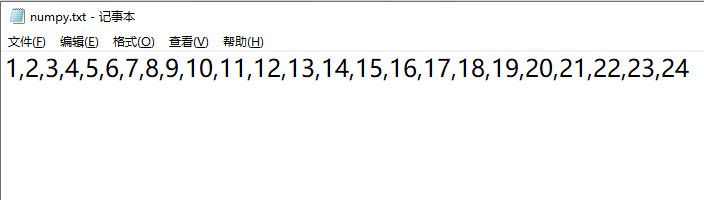# Numpy：数组（Ndarray）操作之数组的转换

### 文章目录

• 数组转换
• 数组转为其他类型
• 数组元素数据类型转换
• 数组的副本和视图
• 概念
• 方法

• 数组操作的内容较多，主要以对数组的转换，数组变化形状，对数组元素进行选择和操作，以及数组元素的增删改查，数组的连接和拆分为主。每种操作都有对应的函数可以实现，需要先掌握这些函数能够实现什么功能，将函数按照功能进行划分。具体使用时从功能模块选择函数，这是最快的学习路径，可以在短时间内掌握对于数组操作的函数。不建议死记硬背函数的语法，这些函数的参数都比较多，而且可选择项也较多，在使用函数的过程中去了解参数，多练习熟能生巧。

# 数组转换

## 数组转为其他类型

方法 说明
ndarray.tolist() 将数组转为列表
ndarray.tostring() 与tobytes具有完全相同的行为
ndarray.tobytes() 构造包含数组中原始数据字节的 Python 字节
ndarray.tofile 将数组作为文本或二进制文件写入文件

ndarray.tolist()

将数组转换为python列表

``````ndarray.tolist()
``````

参数说明：

• 返回值：

• 列表对象
• 示例：

``````>>> a = np.arange(1, 25).reshape(4,6)
>>> a
array([[ 1,  2,  3,  4,  5,  6],
[ 7,  8,  9, 10, 11, 12],
[13, 14, 15, 16, 17, 18],
[19, 20, 21, 22, 23, 24]])
>>> a.tolist() # 将数组转换成为python列表
[[1, 2, 3, 4, 5, 6],
[7, 8, 9, 10, 11, 12],
[13, 14, 15, 16, 17, 18],
[19, 20, 21, 22, 23, 24]]
``````

ndarray.tofile()

将数组以文本或者二进制的格式写入到文件中。

``````ndarray.tofile(fid, sep='', format='%s')
``````

参数说明：

• fid：文件路径和文件名，当文件不存在时可以创建文件。
• sep：接收str，间隔符，默认为空字符串。
• format：文本文件输出的格式字符串。
• 返回值：

• 示例：

``````>>> a = np.arange(1, 25).reshape(4,6)
>>> a
array([[ 1,  2,  3,  4,  5,  6],
[ 7,  8,  9, 10, 11, 12],
[13, 14, 15, 16, 17, 18],
[19, 20, 21, 22, 23, 24]])
>>> a.tofile('numpy.txt', sep='', format='%s')
``````

默认写入文本文件，写入后的格式如图：ndarray.tostring()

与ndarray.tobytes()函数功能一样，将数组转换成二进制字符串。

``````ndarray.tostring(order='C')
``````

参数说明：

• order：{‘C’, ‘F’, ‘A’},可选，默认值为C。
• 返回值：

• 二进制字符串
• 示例：

``````>>> x = np.array([[0, 1], [2, 3]], dtype='<u2')
>>> x
array([[0, 1],
[2, 3]], dtype=uint16)
>>> x.tostring()
b'\x00\x00\x01\x00\x02\x00\x03\x00'
>>> x.tobytes()
b'\x00\x00\x01\x00\x02\x00\x03\x00'
``````

注意：使用tostring()时会有警告提示，如下所示：

``````DeprecationWarning: tostring() is deprecated. Use tobytes() instead.
x.tostring()

``````

但是仍然可以返回结果，推荐使用tobytes()

## 数组元素数据类型转换

方法 说明
ndarray.astype 强制转换数组元素的数据类型为指定类型。
``````ndarray.astype(dtype, order='K', casting='unsafe', subok=True, copy=True)
``````

将数组的数据类型转化为指定的数据类型。

参数：

• dtype：接受字符串或者numpy类型。
• order：可选{‘C’, ‘F’, ‘A’, ‘K’}控制结果的内存布局顺序。
• casting：可选{‘no’, ‘equiv’, ‘safe’, ‘same_kind’, ‘unsafe’}，控制可能发生的数据类型转换。
• ‘no’ 表示根本不应该转换数据类型。
• ‘equiv’ 表示只允许字节顺序更改。
• ‘safe’ 意味着只允许可以保留值的强制转换。
• ‘same_kind’ 表示只允许安全类型转换或类型中的类型转换，如 float64 到 float32。
• ‘unsafe’ 意味着可以进行任何数据转换。
• subok：接收bool值，如果为 True（默认），子类将被传递，否则返回的数组将被强制为基类数组。
• copy：接受bool值，是否赋值数据，默认为True。
• 返回值：

• ndarray
• 示例：

``````>>> import numpy as np
>>> a = np.array([2,4,6,8,10], dtype=np.int32)
array([ 2,  4,  6,  8, 10])
>>> a.dtype # a的数据类型为int32
dtype('int32')
>>> a.astype('int64') # 转换数据类型
array([ 2,  4,  6,  8, 10], dtype=int64)
``````

## 数组的副本和视图

### 概念

副本：是一个数据的完整的拷贝，如果我们对副本进行修改，它不会影响到原始数据，物理内存不在同一位置。

副本一般发生在：

• Python 序列的切片操作，调用deepCopy()函数。
• 调用 ndarray 的 copy() 函数产生一个副本。
• 视图：是数据的一个别称或引用，通过该别称或引用亦便可访问、操作原有数据，但原有数据不会产生拷贝。如果我们对视图进行修改，它会影响到原始数据，物理内存在同一位置。

视图一般发生在：

• numpy 的切片操作返回原数据的视图。
• 调用 ndarray 的 view() 函数产生一个视图。
• ### 方法

以下方法可以创建副本和视图：

方法 说明
ndarray.copy() 返回数组的副本。
ndarray.view() 具有相同数据的数组的新视图。

ndarray.copy()

``````ndarray.copy(order='C')
``````

返回数组的副本。

参数说明：

• order：可选{‘C’, ‘F’, ‘A’, ‘K’},控制副本的内存布局。C为行优先，F为列优先（Fortran）。
• 示例：

``````import numpy as np
x = np.array([[1,2,3],[4,5,6]])
y = x.copy( order='F')
y
``````

输出结果：

``````array([[1, 2, 3],
[4, 5, 6]])
``````

注意：此函数是创建数组副本的首选方法。功能numpy.copy类似，但numpy.copy默认使用顺序’K’，并且默认不会传递子类。

ndarray.view()

返回具有相同数据的数组的新视图。数据与原数组共享。

``````ndarray.view([dtype][, type])
``````

参数说明：

• dtype：可选numpy的数据类型或 ndarray 子类。
• type：可选python数据类型。
• 示例：

``````# 创建数组x
>>> x = np.array([(1, 2),(3,4)], dtype=[('a', np.int8), ('b', np.int8)])
>>> x
array([(1, 2), (3, 4)], dtype=[('a', 'i1'), ('b', 'i1')])
# 创建数组x的视图
>>> y = x.view(dtype=np.int8).reshape(-1,2)
>>> y
array([[1, 2],
[3, 4]], dtype=int8)
``````

对视图进行更改会更改底层数组，示例：

``````>>> y[0,1] = 20 # 更改数组y的元素
>>> x # 数组x的元素也被修改
array([(1, 20), (3,  4)], dtype=[('a', 'i1'), ('b', 'i1')])
``````

当使用ndarray的方法对数组进行操作时，如果运行完后可以直接打印出来内容的一般为创建了视图或者副本。如果运行后没有任何内容打印出来，说明该函数没有生成视图或者副本，而是对原数组进行了操作，可以打印原数组进行查看。理解返回值是什么非常重要。

来源：Mr 李小四